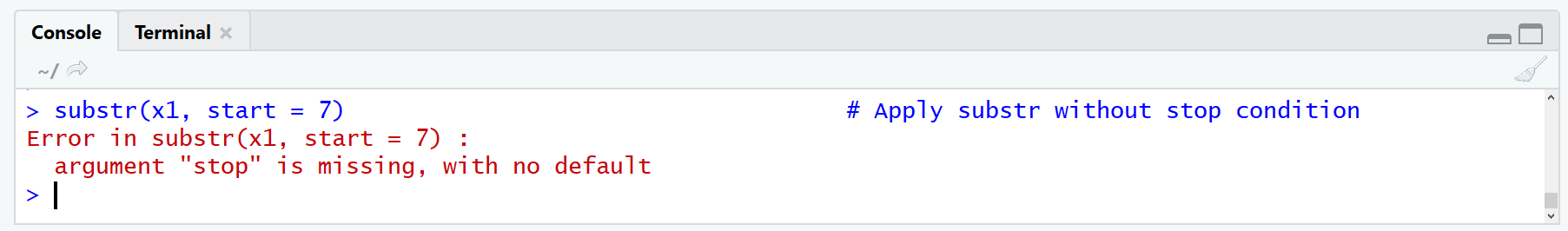# R substr & substring Functions | Examples: Remove, Replace, Match in String

In this R tutorial, I’ll show you how to apply the substr and substring functions. I’ll explain both functions in the same article, since the R syntax and the output of the two functions is very similar. You will learn in which situation you should use which of the two functions.

Basic R Syntax:

```substr(x, start = 2, stop = 5) substring(x, first = 2, last = 5)```

Both, the R substr and substring functions extract or replace substrings in a character vector.

The basic R syntax for the substr and substring functions is illustrated above. In the following R tutorial, I’m going to show you five examples for the usage of substr and substring in the R programming language.

So without further ado, let’s get started…

## Example 1: Extract Substring of Character Vector via substr() & substring()

In the first example, I’m going to show you the probably most popular application of substr and substring: The extraction of some letters of a character vector.

Let’s create such a character vector first:

`x1 <- "hello this is a string" # Create example vector`

Our example vector consists of the simple sentence “hello this is a string”, stored in the character object x1.

We can apply the substr and substring R commands as follows:

```substr(x1, start = 7, stop = 13) # Apply substr # "this is"   substring(x1, first = 7, last = 13) # Apply substring # "this is"```

As you can see, both functions return the same output to the RStudio console: “this is”.

Question: How did we do that?

Answer: Within both functions we specified a starting (i.e. 7) and a finishing point (i.e. 13), between which we extracted all letters of our data object x1.

Note that in case of substr the starting point is called start and the finishing point is called stop; and in case of substring the starting point is called start and the finishing point is called last.

So, if the two functions return the same output, what is actually the difference between substr and substring?

One of these differences, I’m going to show you in Example 2…

## Example 2: Character Vector From Certain Position to the Right

Let’s assume that we want to print everything of our character vector after a certain position. If we remove the stop condition in substr…

`substr(x1, start = 7) # Apply substr without stop condition`

…we get an error:Figure 1: Error in substr: argument “stop” is missing, with no default.

However, if we remove the last option in substring, we get what we want:

```substring(x1, first = 7) # Apply substring without last condition # "this is a string"```

Why does this work?

The reason is that substring has a default value for the last option: last = 1000000L. If we don’t specify last, this value is used. Since our example vector is shorter than 1000000L, the whole rest of the vector after position 7 is printed.

## Example 3: Replace Substring with substr() & substring()

Another popular usage of the substr and substring R functions is the replacement of certain characters in a string.

This is again something we can do with both functions. Let’s first duplicate our example vector twice…

```x2a <- x1 # Duplicate vector for 3rd example x2b <- x1 # Another duplicate```

…and the let’s use substr()…

```substr(x2a, start = 1, stop = 5) <- "heyho" # Replace first word via substr function x2a # "heyho this is a string"```

…and substring()…

```substring(x2b, first = 1) <- "heyho" # Replace first word via substr function x2b # "heyho this is a string"```

…in order to replace “hello” with “heyho”.

Note: The replacement needs to have the same number of characters as the replaced part of your data. If you want to replace a substring with a string with different length, you might have a look at the gsub function.

However, let’s move on to the next example.

## Example 4: Extract Several Substrings

Another difference between substr and substring is the possibility to extract several substrings with one line of code. With substr, this is not possible. If we apply substr to several starting or stopping points, the function uses only the first entry (i.e. the stopping point 1):

```substr(x1, start = 1, stop = 1:5) # Recycling via substr? # "h"```

However, based on the substring R function, it is possible to use several first and last points:

```substring(x1, first = 1, last = 1:5) # Recycling via substring! # "h" "he" "hel" "hell" "hello"```

As you can see, the R substring function returns a vector that contains a substring for each last point that we have specified (i.e. 1, 2, 3, 4 & 5).

Easy peasy!

## Example 5: How to Find Substring Match?

In some situations you might want to know whether a character object contains a certain substring. On the basis of substr and substring, this is unfortunately not (or not easily) possible.

But don’t worry! R has many other functions that can be used for this task. Even though this tutorial is about substr and substring, you may want to know how to check whether a substring exists within a string.

For this task, we can use the grepl function. With the following R code, we can find the character pattern “hello” in our example vector x1:

```grepl("hello", x1) # Find substring via grepl # TRUE```

We got a match – our example vector x1 contains the word “hello”.

```grepl("hello", x2a) # No substring match found # FALSE```

You guessed it, grepl returns FALSE.

## Video: Further Examples for substr()

Sometimes it’s easier to understand something, when it is visualized in a video. If you need further explanations and examples on how to remove, find, or replace a substring in R, I can recommend the following video of Jonatan Lindh’s YouTube channel.